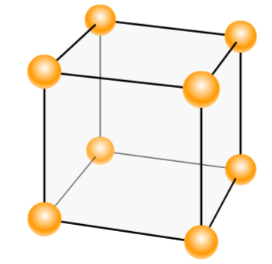# Problem: Consider a simple cubic (a.k.a, primitive cubic) unit cell as shown here.(i) How many atoms are shown in this image?(ii) What fraction of each atom is inside the boundaries of the cube?(iii) If you sum all (the fractions of atoms, how many atoms are actually inside a simple cubic unit cell?

🤓 Based on our data, we think this question is relevant for Professor Wingrave's class at UD.

###### FREE Expert Solution###### Problem Details

Consider a simple cubic (a.k.a, primitive cubic) unit cell as shown here.

(i) How many atoms are shown in this image?

(ii) What fraction of each atom is inside the boundaries of the cube?

(iii) If you sum all (the fractions of atoms, how many atoms are actually inside a simple cubic unit cell?What scientific concept do you need to know in order to solve this problem?

Our tutors have indicated that to solve this problem you will need to apply the Unit Cell concept. You can view video lessons to learn Unit Cell. Or if you need more Unit Cell practice, you can also practice Unit Cell practice problems.

What is the difficulty of this problem?

Our tutors rated the difficulty ofConsider a simple cubic (a.k.a, primitive cubic) unit cell a...as medium difficulty.

How long does this problem take to solve?

Our expert Chemistry tutor, Dasha took 1 minute and 45 seconds to solve this problem. You can follow their steps in the video explanation above.

What professor is this problem relevant for?

Based on our data, we think this problem is relevant for Professor Wingrave's class at UD.